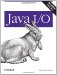How Object Serialization Works

Objects possess state. This state is stored in the values of the nonstatic, nontransient fields of an object's class. Consider this TwoDPoint class:

public class TwoDPoint {
public double x;
public double y;
}

Every object of this class has a state defined by the values of the double fields x and y. If you know the values of those fields, you know the value of the TwoDPoint. Nothing changes if you add some methods to the class or make the fields private, as in Example 13-1.

Example 13-1. The TwoDPoint class

 public class TwoDPoint { private double x; private double y; public TwoDPoint(double x, double y) { this.x = x; this.y = y; } public double getX( ) { return x; } public double getY( ) { return y; } public String toString( ) { return "[TwoDPoint:x=" + this.x + ", y=" + y +"]"; } }

The object state, the information stored in the fields, is still the same. If you know the values of x and y, you know everything there is to know about the state of the object. The methods only affect the actions an object can perform. They do not change what an object is.

Now suppose you wanted to save the state of a particular TwoDPoint object by writing a sequence of bytes onto a stream. This process is called serialization since the object is serialized into a sequence of bytes. You could add a writeState( ) method to your class that looked something like this:

public void writeState(OutputStream out) throws IOException {
DataOutputStream dout = new DataOutputStream(out);
dout.writeDouble(x);
dout.writeDouble(y);
}

To restore the state of a Point object, you could add a readState( ) method like this:

public void readState(InputStream in) throws IOException {
DataInputStream din = new DataInputStream(in);
this.x = din.readDouble( );
this.y = din.readDouble( );
}

Needless to say, this is a lot of work. You would have to define readState( ) and writeState( ) methods for every class whose instances you wanted to serialize. Furthermore, you would have to track where in the byte stream particular values were stored to make sure that you didn't accidentally read the y coordinate of one point as the x coordinate of the next. You'd also have to make sure you could serialize the object's superclasses if the superclass contained a relevant state. Classes composed of other classes would cause a lot of trouble since you'd need to serialize each object the first object contained, then each object those objects contained, then the objects those objects contained, and so forth. Finally, you'd need to avoid circular references that could put you in an infinite loop.

Fortunately, Sun's done all the work for you. Object streams can access all the nonstatic, nontransient fields of an object (including the private parts) and write them out in a well-specified format. All you have to do is chain object output streams to an underlying stream where you want the object to be written and call writeObject( ); you do not have to add any new methods. Reading objects in from an object input stream is only slightly more complicated; in addition to reading the object from the stream, you also need to cast the object to the correct type.Java I/O
ISBN: 0596527500
EAN: 2147483647
Year: 2004
Pages: 244

Similar book on Amazon